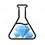# LaTeX

Does anyone reading this know how to,

-> Make colored text?

-> Make outlines of words?

-> Type in Italics?Note by I Love Brilliant
1 year ago

This discussion board is a place to discuss our Daily Challenges and the math and science related to those challenges. Explanations are more than just a solution — they should explain the steps and thinking strategies that you used to obtain the solution. Comments should further the discussion of math and science.

When posting on Brilliant:

• Use the emojis to react to an explanation, whether you're congratulating a job well done , or just really confused .
• Ask specific questions about the challenge or the steps in somebody's explanation. Well-posed questions can add a lot to the discussion, but posting "I don't understand!" doesn't help anyone.
• Try to contribute something new to the discussion, whether it is an extension, generalization or other idea related to the challenge.

MarkdownAppears as
*italics* or _italics_ italics
**bold** or __bold__ bold
- bulleted- list
• bulleted
• list
1. numbered2. list
1. numbered
2. list
Note: you must add a full line of space before and after lists for them to show up correctly
paragraph 1paragraph 2

paragraph 1

paragraph 2

[example link](https://brilliant.org)example link
> This is a quote
This is a quote
    # I indented these lines
# 4 spaces, and now they show
# up as a code block.

print "hello world"
# I indented these lines
# 4 spaces, and now they show
# up as a code block.

print "hello world"
MathAppears as
Remember to wrap math in $$ ... $$ or $ ... $ to ensure proper formatting.
2 \times 3 $2 \times 3$
2^{34} $2^{34}$
a_{i-1} $a_{i-1}$
\frac{2}{3} $\frac{2}{3}$
\sqrt{2} $\sqrt{2}$
\sum_{i=1}^3 $\sum_{i=1}^3$
\sin \theta $\sin \theta$
\boxed{123} $\boxed{123}$

Sort by:

Check @Hamza Anushath's LaTeX guide.

- 1 year ago

Thnx @Yajat Shamji!

- 1 year ago

$\text{Just like how}$ @Yajat Shamji $\text{said, you should better check my guide.}$

- 1 year ago

• Use $\LaTeX$ and type in the function \color{colorname}{whatever}
• Again in $\LaTeX$ use \boxed{text}
• To type in italics use 1 asterisk on either side, for example like this

- 1 year ago

there's no spaces!! :\

- 1 year ago

Where? I don't understand

- 1 year ago

thank you!!!!! :)

- 1 year ago

• $\color{#3D99F6} This \ is \ colored \ text$

• $\mathbb{IS \ THIS \ GOOD \ ENOUGH \ FOR \ YOU?}$

• $\textsf{Which}$ $\texttt{one}$ $\textit{do}$ $\mathcal{you}$ $\mathfrak{like?}$

.

.

Mahdi Raza already gave color so I'll give the other codes @I Love Brilliant :)

• \mathbb{IS \ THIS \ GOOD \ ENOUGH \ FOR \ YOU?}\

• \textsf{Which} \texttt{one} \textit{do} \mathcal{you} \mathfrak{like?}